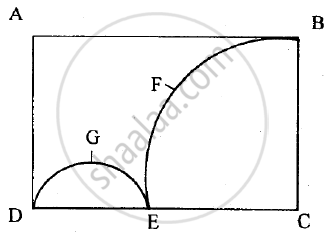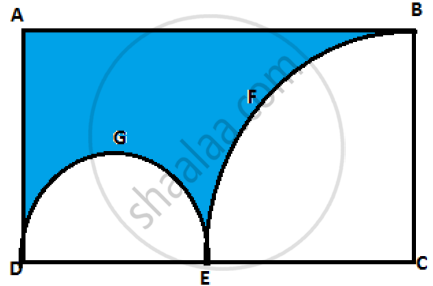Share

# In the Figure Given Below, Abcd is the Rectangle. Ab = 14 Cm, Bc = 7 Cm. from the Rectangle, a Quarter Circle Bfec and a Semicircle Dge Are Removed. Calculate the Area of the Remaining Piece of the Rectangle. (Take Pi = 22/7) - ICSE Class 10 - Mathematics

ConceptCircle - Direct Application Problems Including Inner and Outer Area

#### Question

In the figure given below, ABCD is the rectangle. AB = 14 cm, BC = 7 cm. From the rectangle, a quarter circle BFEC and a semicircle DGE are removed. Calculate the area of the remaining piece of the rectangle. (Take pi = 22/7).#### Solution

Considering the given figure:Given dimensions of the rectangle: AB = 14 cm and BC =  7 cm

⇒ Radius of the quarter circle = 7 cm

Area of the quarter circle = 1/2 xx 22/7 xx 7^2 sq. cm = 77/2 sq. cm

Since EC = 7 cm and DC = 14 cm, we have

Therefore, radius of the semi circle =  7/2 cm

=> Area of the semi circle = 1/2 xx 22/7 xx (7/2)^2 sq. cm = 77/4 sq. cm

Now, are of  rectangle ABCD = AB x BC = 14 x 7 = 98 sq. cm

∴ Required area =  Area of rectangle ABCD - [Area(BCEF) + Area(DGE)]

= 98 - 77/2 - 77/4

= 161/4

= 40.25 sq. cm

Is there an error in this question or solution?

#### APPEARS IN

2013-2014 (March) (with solutions)
Question 3.3 | 4.00 marks
Solution In the Figure Given Below, Abcd is the Rectangle. Ab = 14 Cm, Bc = 7 Cm. from the Rectangle, a Quarter Circle Bfec and a Semicircle Dge Are Removed. Calculate the Area of the Remaining Piece of the Rectangle. (Take Pi = 22/7) Concept: Circle - Direct Application Problems Including Inner and Outer Area.
S